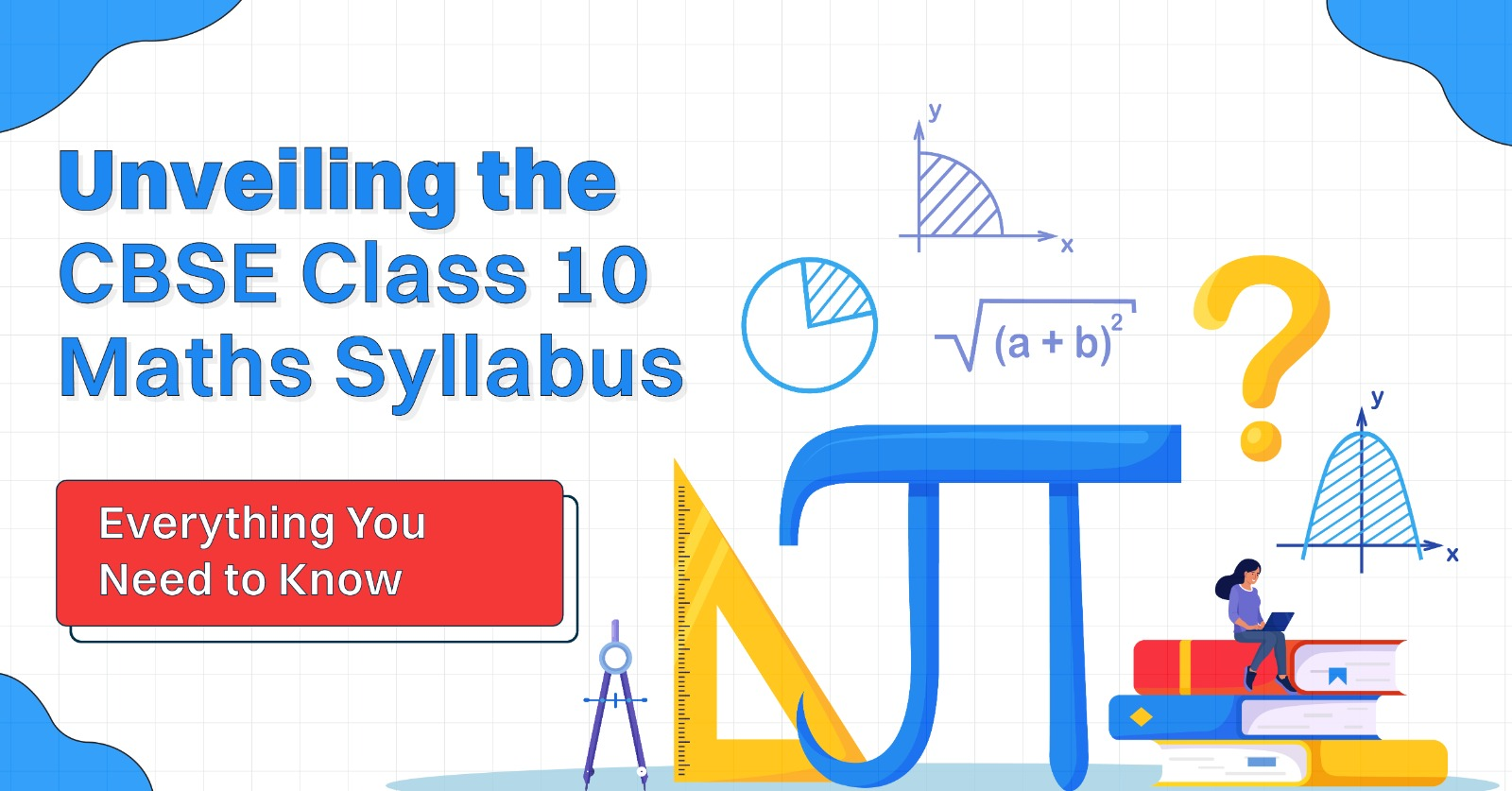# “Unveiling the CBSE Class 10 Maths Syllabus: Everything You Need to Know"

Anyone in the worldCBSE Class 10 Maths Syllabus is one of the most crucial subjects for students. The syllabus covers a vast range of topics that provide a strong foundation for higher education. In this blog, we will discuss the CBSE Class 10 Maths Syllabus in detail, along with NCERT Books Class 10 Maths and preparation tips.

CBSE Class 10 Maths Syllabus

The CBSE Class 10 Maths Syllabus comprises five units: Number Systems, Algebra, Geometry, Trigonometry, and Statistics & Probability. Here's a detailed look at each unit:

Unit 1: Number Systems The first unit of the CBSE Class 10 Maths Syllabus is Number Systems, which focuses on the concepts of real numbers, rational numbers, irrational numbers, laws of exponents, and the decimal representation of rational numbers.

Unit 2: Algebra The second unit of the CBSE Class 10 Maths Syllabus is Algebra, which includes the study of linear equations in two variables, quadratic equations, arithmetic progressions, and polynomials.

Unit 3: Coordinate Geometry The third unit of the CBSE Class 10 Maths Syllabus is Coordinate Geometry, which covers the concept of geometry using coordinates. This includes the distance formula, section formula, and the area of a triangle.

Unit 4: Geometry The fourth unit of the CBSE Class 10 Maths Syllabus is Geometry, which involves the study of triangles, circles, constructions, and areas related to circles.

Unit 5: Trigonometry The fifth unit of the CBSE Class 10 Maths Syllabus is Trigonometry, which covers the study of trigonometric ratios, trigonometric identities, and the application of trigonometry in solving real-world problems.

The CBSE Class 10 Maths Syllabus includes 80 marks for theory and 20 marks for practicals. The theory portion consists of two parts - internal assessment (20 marks) and external examination (60 marks). The internal assessment includes periodic tests, assignments, and projects, while the external examination is conducted by the CBSE.

NCERT Books Class 10 Maths

NCERT Books Class 10 Maths are essential for CBSE Class 10 students. NCERT provides well-structured and easy-to-understand textbooks for students. These books help students learn the concepts effectively and include several practice questions.

Preparation Tips

1. Understand the concepts: Start by understanding the concepts thoroughly. This will help you to solve problems quickly and effectively.

2. Practice: Practice is the key to success. Make sure you practice as many questions as possible to improve your speed and accuracy.

3. Solve previous year papers: Solving previous year papers will help you to understand the exam pattern and the type of questions that are asked.

5. Time management: Time management is crucial during the exam. Make sure you manage your time well during the exam.

Previous Year Question Papers Class 10 Maths For 2023 Exam – Click Here